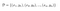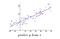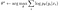# From self-attention to transformers

In a previous article, I have talked about how we can build sequence to sequence models and improve their ability to handle long-range dependencies by using an attentional mechanism.

Here, we’re going to talk about another class of models for processing sequences that does not use recurrent connections, but instead relies entirely on attention. We’ll build towards a class of models called transformers.

The basic question is: can we get away with using attentional connections without any explicit recurrence? In principle, attention can grab any information you want from the input string and our RNN can be transformed into a…

# Sequence to sequence models

Knowledge about RNNs is a prerequisite to understand this article. In case you don’t know RNNs, check out my article.

We’re going to talk about how we can use recurrent neural networks to solve some interesting problems; we will focus on how we can train and utilize sequence to sequence models.

Recurrent neural networks are very flexible and can be used to solve a wide range of different types of sequence processing problems:

In this article, we’re going to focus on the many to many problems. Before that, let’s discuss how we can build a basic neural language model, which…

# Evaluation of Reinforcement Learning Policies

## In reinforcement learning, the agent usually deals with feedback that is sequential, evaluative and sampled.

In this article, we will focus on agents that can deal with feedback that’s simultaneously sequential and evaluative. And even most humans have problems with simultaneously balancing immediate and long-term goals and the gathering and utilization of information.

• Sequential means that your agents can receive delayed information. Delayed feedback makes it tricky to interpret the source of the feedback. Sequential feedback gives rise to the temporal credit assignment problem, which is the challenge of determining which state, action, or state-action pair is responsible for a reward.
• Evaluative means that the feedback is only relative, because the environment is uncertain. We…

# Recurrent neural networks

What if we have a variable size input?

A sequence input can be of variable length. For example, they might be sentences in English (the first sequence has four elements, the second one has three elements and the third one has five elements):

This could involve:

• Sequence of words → The problem of sentiment classification: you have a review online and you want to guess whether this is a positive or a negative review.
• Sequence of sounds → The problem of recognizing a phoneme from a sound
• Sequence of images → The problem of classifying the activity in a video

# Basic concepts in machine learning

Machine learning algorithms try to imitate the pattern between two datasets in such a way that they can use one dataset to predict the other. Specifically, supervised machine learning is useful for taking what you know as input and quickly transforming it into what you want to know.

In order to explain the basics of supervised learning, I will first start with some basic probability theory. Afterwards, I will describe the “machine learning model”.

# Some probability theory

Let’s define supervised learning a little bit more precisely. We’re going to assume that during training we are given a dataset:Training set consisting of tuples; the y’s are the true labels corresponding to the x’s.

For example, if we wanted…

# The chain rule of calculus

Neural networks consist of compositions of many functions. Taking derivatives through compositions of functions is what the chain rule of calculus helps us figure out.

If you have the expression:

That means you have an input x, you apply a function g to x, you get y and you apply another function f to y. Eventually, you get z.

# Error Analysis in Deep Learning

Let’s try to mathematically understand what overfitting and underfitting are. In order to do so, we’ll focus on regression — this means that you are predicting a continuous variable or a continuous distribution based on your input.

Regression is basically curve fitting:Taken from Levine, CS182, 2021.

You have some continuous or discrete inputs and you have a continuous output and you want to predict that output. For example, you’re predicting how big the dog is, how much it weighs, how expensive the house is, and so on.

If we want to adopt a probabilistic approach, in order to do regression we need a probability…

# Optimization in deep learning

We’re going to talk about optimization algorithms. Let’s start with a recap of gradient descent.

We can formulate the problem of learning θ as our attempt to find the θ that maximizes the log p(D):This is called maximum likelihood estimation (MLE), because this log probability is the likelihood and we are choosing θ to maximize the likelihood

Often times, we will see this written as a minimization (this is just a convention):

# Convolutional neural networks

Let’s say you have the picture of a cute puppy. You want to classify whether it’s a picture of a puppy, of a cat, of a hippopotamus or of a giraffe.

You could train a fully connected network to solve this task:

# Backpropagation step by step

To figure out how to get a complete learning algorithm with neural networks, we have to remember the steps for solving any machine learning problem:

1 Define your model class

2 Define your loss function

3 Pick your optimizer

Regarding point 3, we need to figure out how to find the setting of θ that minimizes our loss function. I find it useful to think about the concept of loss landscape. You can think of the loss landscape as a plot of the loss function. L(θ) denotes the loss function.

Let’s say that θ is 2D: you could imagine a…## Samuele Bolotta

PhD student in Machine Learning — also interested in Neuroscience and Cognitive Science.